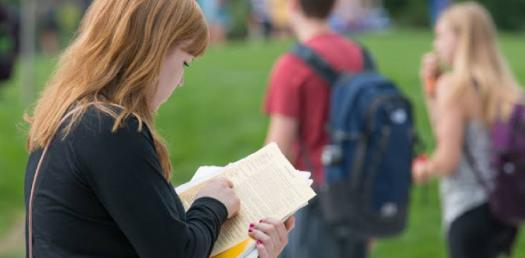# AP Statistics: Test Your Knowledge! Trivia Quiz

5 Questions | Attempts: 784
ShareSettings.

• 1.
Data were collected on two variables X and Y and a least-squares regression line was fitted to the data. The estimated equation for this data is y = -2.29 + 1.70x. One point has x = 5 and y = 6. What is the residual for this point?
• A.

7.91

• B.

6.21

• C.

0.21

• D.

-0.21

• E.

-2.91

• 2.
In a clinic, 50 patients with sleep disorders are randomly assigned to one of two different groups.  Patients in one group are given medication before bedtime.  Patients in another group are given blindfolds and played soft music at bedtime.  Each patient is attached to a machine that records breathing patterns.  From the patterns, it is possible to determine if the patient is awake or asleep.  The data will be used to decide which method is more effective in helping patients with sleep disorders.  Which of the following statements is correct in the context of this experiment?
• A.

This is a double blind experiment because the patients were divided into the two treatment groups randomly.

• B.

It is not possible for this experiment to be single-blind or double-blind, because human subjects are involved.

• C.

This is only a single-blind experiment because the experimenters did not include a control group that received neither medication nor a blindfold and soft music.

• D.

This is only a single-blind experiment because patients will know which treatment they are receiving, although the examining doctor might not.

• E.

This is a double-blind experiment because the experimenters could prevent both groups of patients from knowing what sort of treatment the other group is receiving.

• 3.
In which of the following situations is a binomial model not an appropriate model to describe the outcome?
• A.

The number of heads in three tosses of a coin.

• B.

The number of rainy days in a given week.

• C.

The number of girls in a randomly selected family of five children.

• D.

The number of students over five feet tall at a randomly selected middle school.

• E.

The number of defective computer parts randomly selected from an assembly line.

• 4.
• A.

A

• B.

B

• C.

C

• D.

D

• E.

E

• 5.
Two random variables, X and Y, are independent.  X has an expected value 2.5 and a standard deviation 0.3, while Y has an expected value 4.7 and standard deviation 0.4.  Which of the following is true?
• A.

The mean of X + Y is 6.2.

• B.

The standard deviation of X + Y is 0.7.

• C.

The variance of X + Y is 0.7.

• D.

The standard deviation of X - Y = 0.1

• E.

The standard deviation of X - Y = 0.5

## Related TopicsBack to top
×

Wait!
Here's an interesting quiz for you.